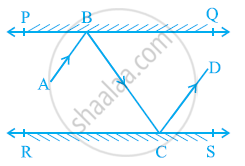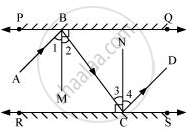# In the given figure, PQ and RS are two mirrors placed parallel to each other. An incident ray AB strikes the mirror PQ at B, the reflected ray moves along the path BC and strikes the mirror RS at C and again reflects back along CD. Prove that AB || CD. - Mathematics

In the given figure, PQ and RS are two mirrors placed parallel to each other. An incident ray AB strikes the mirror PQ at B, the reflected ray moves along the path BC and strikes the mirror RS at C and again reflects back along CD. Prove that AB || CD.#### SolutionLet us draw BM ⊥ PQ and CN ⊥ RS.

As PQ || RS,

Therefore, BM || CN

Thus, BM and CN are two parallel lines and a transversal line BC cuts them at B and C respectively.

∴∠2 = ∠3 (Alternate interior angles)

However, ∠1 = ∠2 and ∠3 = ∠4 (By laws of reflection)

∴ ∠1 = ∠2 = ∠3 = ∠4

Also, ∠1 + ∠2 = ∠3 + ∠4

∠ABC = ∠DCB

However, these are alternate interior angles.

∴ AB || CD

Concept: Lines Parallel to the Same Line
Is there an error in this question or solution?
Chapter 6: Lines and Angles - Exercise 6.2 [Page 105]

#### APPEARS IN

NCERT Class 9 Maths
Chapter 6 Lines and Angles
Exercise 6.2 | Q 6 | Page 105

Share